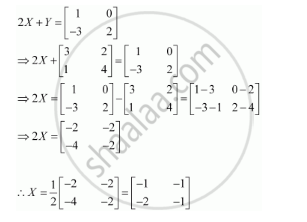Share

# Find X, If Y = [(3, 2),(1,4)] and 2x + Y = [(1, 0),(-3, 2)] - CBSE (Commerce) Class 12 - Mathematics

ConceptOperations on Matrices Properties of Scalar Multiplication of a Matrix

#### Question

Find X, if  Y = [(3, 2),(1,4)] and 2X + Y = [(1, 0),(-3, 2)]

#### SolutionIs there an error in this question or solution?

#### APPEARS IN

Solution Find X, If Y = [(3, 2),(1,4)] and 2x + Y = [(1, 0),(-3, 2)] Concept: Operations on Matrices - Properties of Scalar Multiplication of a Matrix.
S